All Ruby on Rails Node JS Android iOS React Native Frontend Flutter QA

# Runtime of Algorithms Based on the Input Size

Have you ever wondered how will your code scale up or how runtime of your function will change depending on the input size? If yes, you are in the right place and in the next few minutes, you will learn how to look at your code in terms of performance and scalability.

## Big O notation

To visualize how our code scales up, we will use Big O notation, which is a notation that shows the limiting behavior of a function when the argument tends towards a particular value or infinity. In essence, it will show us how our algorithm performs when its input size gets larger. For example, if we increase the size of an array from 5 to 100 elements will the runtime of a function:

• be constant,
• get proportionally larger,
• get exponentially larger,
• change in some other way?

## Constant runtime

A constant runtime means that regardless of the size of the input we provide to a function, its time complexity will stay the same. We call this behavior O(1).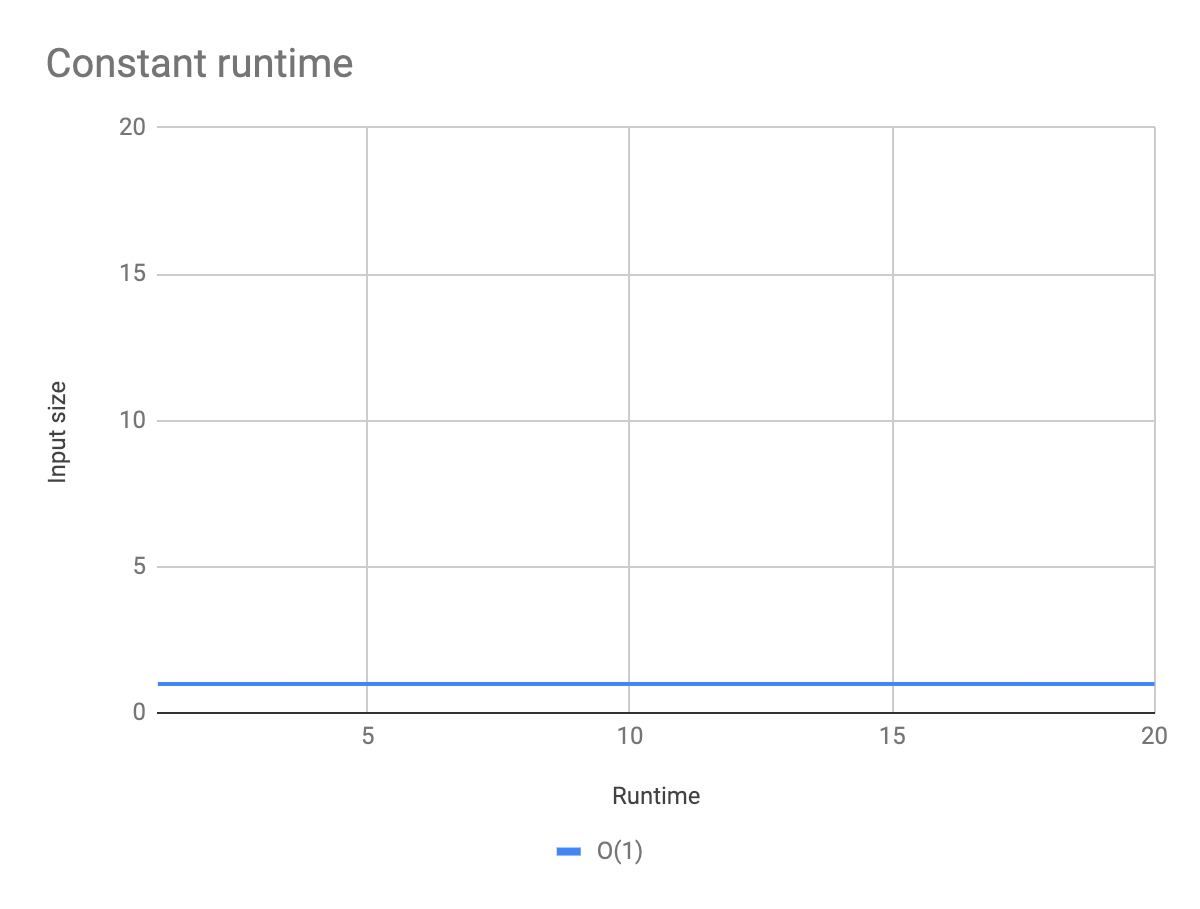To present an algorithm with a constant runtime, I created a simple function that console-logs the first two elements of an array. You can see an example of the code below.

``````
function constantRuntime(array) {
console.log(array);
console.log(array);
}

constantRuntime([1,2,3]); // 2 operations
constantRuntime([1,2,3,4,5,6,7,8,9,10]); // 2 operations
```
```

In both cases of calling the function, the number of operations will be two. These types of functions can be very efficient and their time complexity doesn’t increase with the size of the input.

## Linear runtime

In this case, our runtime will increase proportionally to how much our input increases, and we mark it as O(n).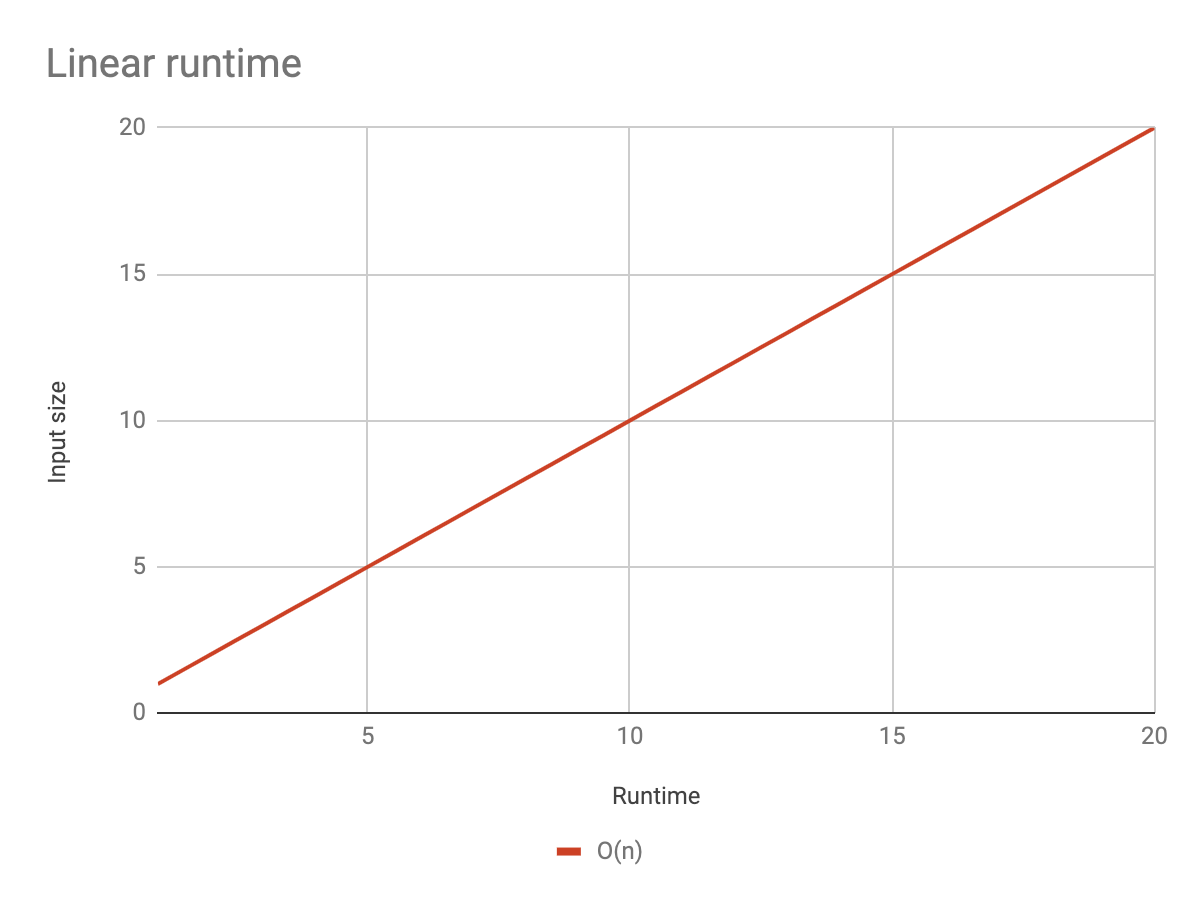I made a simple function that loops over the given array and console-logs every element of an array.

``````
function linearRuntime(array) {
array.forEach(item => console.log(item));
}

linearRuntime([1,2,3]); // 3 operations
linearRuntime([1,2,3,4,5,6,7,8,9,10]); // 10 operations
```
```

As you can see above, the number of operations increased linearly with the size of the input. These types of functions are efficient, and in most cases, there’s no better way than to loop over the whole array. But still, in cases such as searching for an element in an array, they are commonly overused, and there are better ways to achieve the same results. One of them is binary search, which you will learn about in a second.

## Exponential runtime

In this example, the function’s runtime will increase exponentially, which means it will increase 2^(input size). In terms of Big O, notation this is called O(n^2).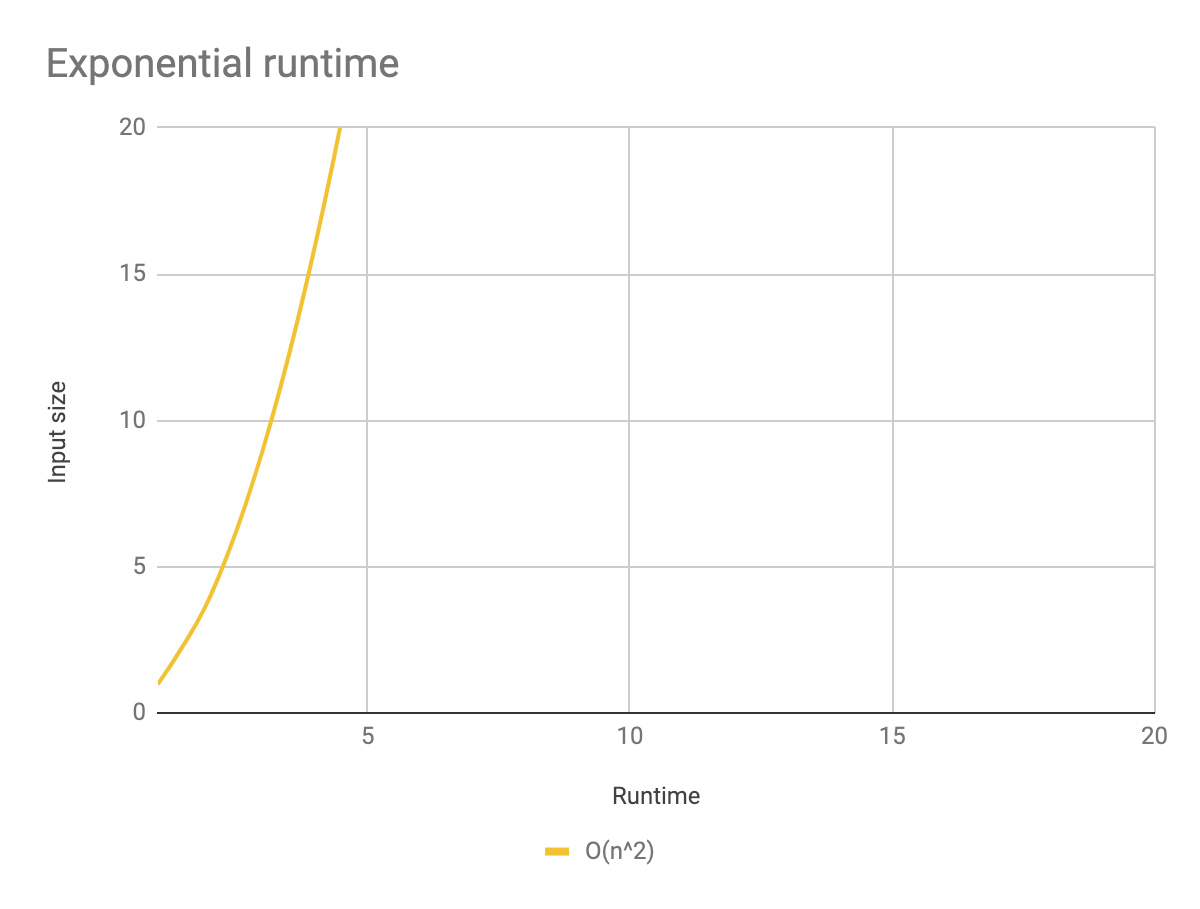To show an algorithm with an exponential runtime, I made a function that creates all possible pairs from a given array, for example, 11, 12, 13, 21, 22 etc. as you can see below.

``````
function exponentialRuntime(array) {
array.forEach(itemOne => {
array.forEach(itemTwo => {
console.log(`\${itemOne}\${itemTwo}`);
})
})
}

exponentialRuntime([1,2,3]); // 9 operations
exponentialRuntime([1,2,3,4,5,6,7,8,9,10]); // 100 operations
```
```

For very small inputs, you probably won’t notice any performance issues but these types of functions get out of hand very easily and as your input grows – functions with an exponential runtime are getting really inefficient and can slow down your application. You should avoid using them or use them very cautiously. As you can see in the second function call, we get 100 operations from an array that has only 10 elements!

## Logarithmic runtime

Functions with a logarithmic runtime are the inversion of an exponential function. In terms of Big O notation, we call these functions O(log n).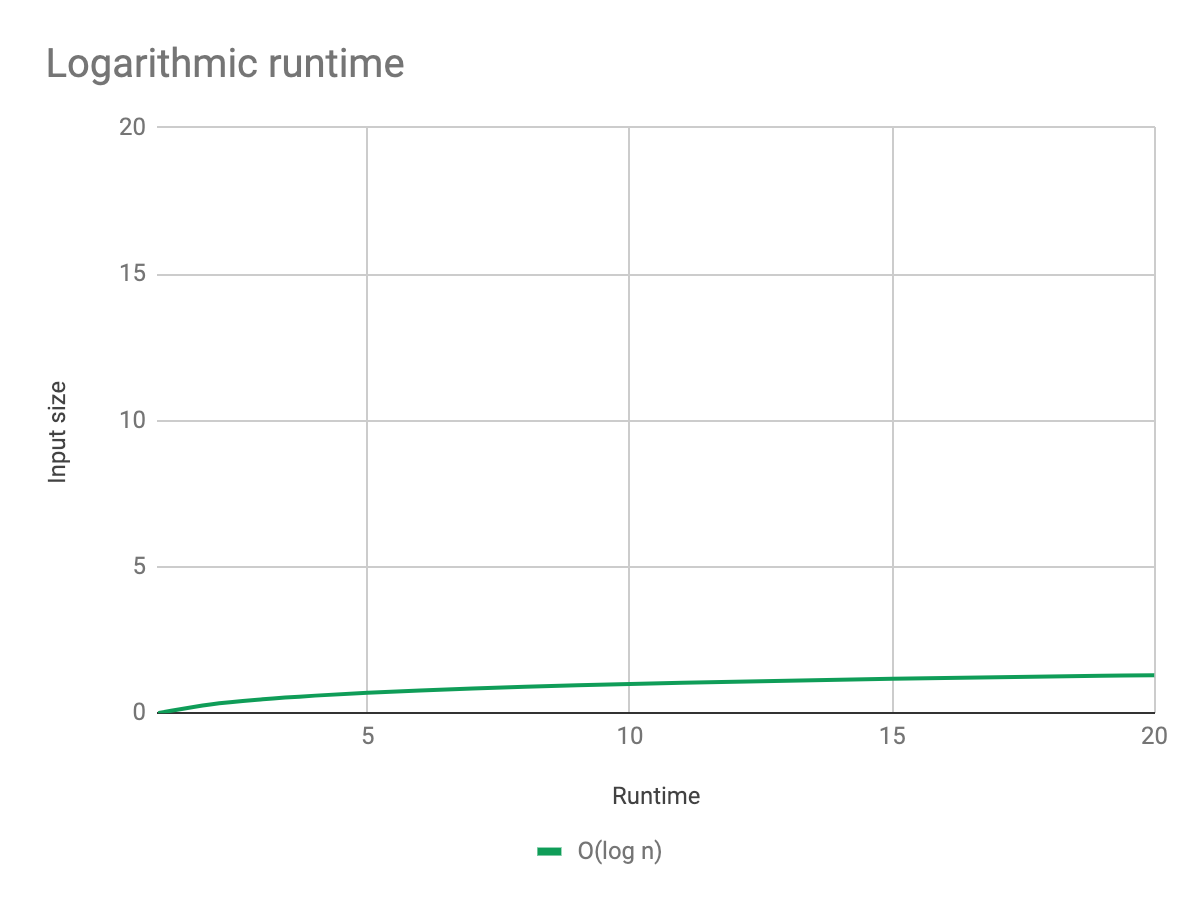I created a function that represents a binary search. Binary search accepts two parameters: an array that is sorted in some way, (in my example, it was sorted in the ascending order), and a key value to search within our array. You can regard binary search as if you were searching for word in a dictionary. If you want to find a single word, for example, that starts with a letter P, you will open the dictionary in the middle and find out that you are in the section with the letter M. You know that letter P is further in an alphabet, so you can forget about the first half of a dictionary. By doing it, we are cutting our input in half and we are left with a range of letters from M to Z. So now, we jump into the middle of our range, and we will find the letter S. We know that P is before S, so we are left with the range of letters M to S. Once again, we open this section in the middle, and voilà!, we are in the section with the letter P. This is how binary search works, and its logarithmic runtime derives from cutting the input in a half.

``````
function logarithmicRuntime(array, key) {
let low = 0;
let high = array.length - 1;
let mid, element;

while (low <= high) {
console.log('o');
mid = Math.floor((low + high) / 2);
element = array[mid];

if (element < key) {
low = mid + 1;
} else if (element > key) {
high = mid - 1;
} else {
return mid;
}
}
return -1;
}

logarithmicRuntime([1,2,3], 3); // 2 operations
logarithmicRuntime([1,2,3,4,5,6,7,8,9,10], 8); // 2 operations
```
```

You might have noticed we found the letter P within only 3 operations, which is really powerful. In comparison to a standard function with a linear runtime, it would need over 16 operations to get to the destination point. If we were to go further and increase the size of the array to 4000 elements, a binary search would take only about 12 operations to find every element we are looking for. Functions with a logarithmic runtime scale very well, and we definitely should use them if we have a lot of data.

## Summary

If we compare all the above types of functions, we will clearly see in the graph below that functions with a logarithmic runtime are almost as efficient as functions with a static runtime.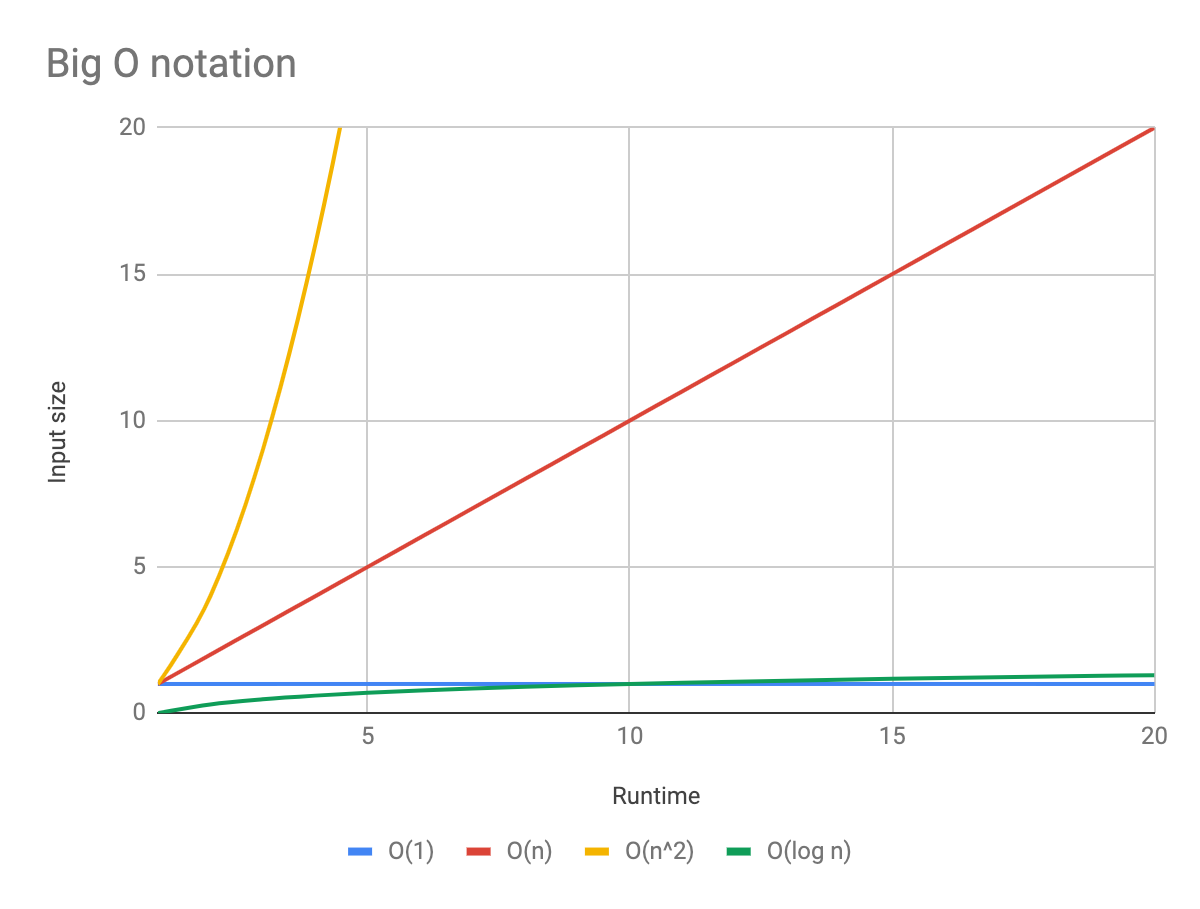Without a doubt, we shouldn’t start using binary search to look through an array of 5 elements to get a single value. But when it comes to big data, we should be aware of what we are doing and check if we are not drastically slowing down our application.